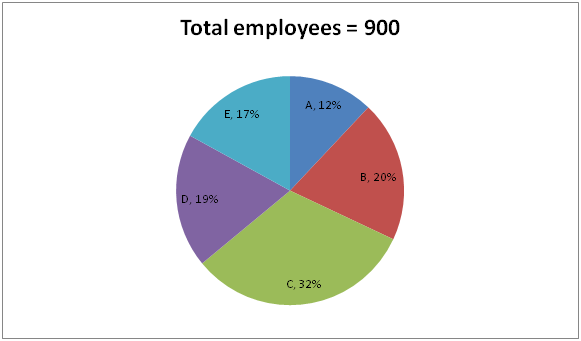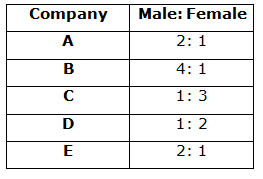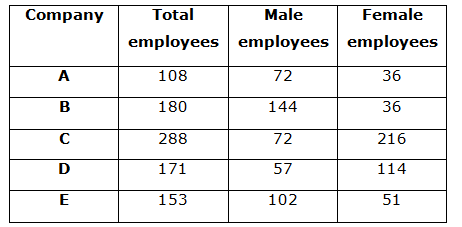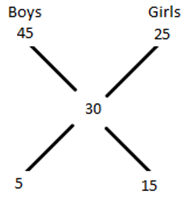# SBI Clerk Prelims 2021 Quantitative Aptitude Questions (Day-22)

Dear Aspirants, Our IBPS Guide team is providing new series of Quantitative Aptitude Questions for SBI Clerk Prelims 2020 so the aspirants can practice it on a daily basis. These questions are framed by our skilled experts after understanding your needs thoroughly. Aspirants can practice these new series questions daily to familiarize with the exact exam pattern and make your preparation effective.

Start Quiz

Pie chart

Directions (01-05): Study the following information carefully and answer the questions given below.

The given pie chart shows the percentage of the total number of employees in five different companies.The given table shows the ratio of the number of male to female employees in that companies.1) Find the total female employees in all the companies together?

A.453

B.456

C.450

D.448

E.None of these

2) What is the difference between the number of male employees from C and the number of female employees from B?

A.30

B.32

C.36

D.28

E.24

3) Total number of male employees from E is approximately what percent more than the number of male employees from D?

A.75%

B.77%

C.79%

D.81%

E.73%

4) The total number of male employees from A, B and C together is what percent of the total number of employees from A, B and C together?

A.45%

B.48%

C.36%

D.50%

E.55%

5) In company F, the number of male employees is 25% more than the number of male employees from B and the number of female employees in F is 25% more than the number of female employees from A. What is the ratio of the total number of employees from F to C?

A.25: 32

B.24: 31

C.26: 33

D.31: 26

E.None of these

Average

6) Average weight of the boys in the class is 45 kg and average weight of the girls in the class is 25 kg. If the average weight of the class is 30 kg, then find the ratio of the number of boys to girls in the class?

A.1: 4

B.2: 3

C.2: 1

D.1: 3

E.None of these

Age

7) Average ages of Arun and Anu is 30 years. If the double of the sum of their age is six times Anu’s age, then what is the present age of Arun?

A.30 years

B.40 years

C.35 years

D.45 years

E.None of these

Mensuration

8) If the area of a rectangle is 432 cm2 and the length of the rectangle is 6 cm more than the breadth of the rectangle. If the radius of the circle is 4 cm less than the breadth of the rectangle, then what is the difference between the perimeter of the rectangle and circumference of the circle?

A.4 cm

B.6 cm

C.8 cm

D.10 cm

E.12 cm

Time and work

9) A alone complete the work in 24 days and A and B together can complete the work in 16 days. If A started the work and after 8 days left, then B alone complete the remaining work, in how many days, B complete the remaining work?

A.24 days

B.28 days

C.30 days

D.32 days

E.None of these

Ratio and proportion

10) If the number of male employees in A is 40% more than the number of female employees from A and the number of female employees in B is 40% less than the number of male employees in B. If the total number of employees in A is 50% more than the total number of employees in B, then what is the ratio of the number of male to female employees from A and B together?

A.2: 5

B.3: 2

C.2: 1

D.1: 3

E.3: 4

Directions (1-5):Total number of female employees = 36 + 36 + 216 + 114 + 51 = 453

Required difference = 72 – 36 = 36

Required percentage = (102 – 57)/57 * 100 = 79%

Required percentage = (72 + 144 + 72)/(108 + 180 + 288) * 100

= 50%

Number of male employees from F = 125/100 * 144 = 180

Number of female employees from F = 125/100 * 36 = 45

Total number of employees from F = 180 + 45 = 225

Required ratio = 225: 288

= 25: 32Required ratio = 5: 15 = 1: 3

Arun + Anu = 30 * 2 = 60

2 * 60 = 6 * Anu

Anu = 20 years

Arun = 60 – 20 = 40 years

Area of the rectangle = 432

Length of the rectangle = x

Breadth of the rectangle = x – 6

x * (x – 6) = 432

x2 – 6x – 432 = 0

x2 – 24x + 18x – 432 = 0

x(x – 24) + 18(x – 24) = 0

x = 24, -18 (negative value is neglect)

Breadth of the rectangle = 24 – 6 = 18 cm

Perimeter of the rectangle = 2 * (18 + 24) = 84 cm

Radius of the circle = 18 – 4 = 14 cm

Circumference of the circle = 2 * 22/7 * 14 = 88 cm

Required difference = 88 – 84 = 4 cm

LCM of 24 and 16 = 48

Total work = 48 units

A’s per day work = 2 units

(A + B)’s per day work = 3 units

B’s per day work = 1 unit

A’s 8 days work = 16 units

Remaining work = 48 – 16 = 32

Required no. of days = 32/1 = 32 days

(Or)

A = 1/24

A + B = 1/16

B = 1/16 – 1/24 = 1/48

A works for 8 days complete 8/24 of the work.

Remaining work = 2/3

B alone complete 2/3 of the work = 48 * 2/3 = 32 days

Number of employees in B = x

Number of employees in A = x * 150/100 = 3x/2

Number of male to female employees in A = 140: 100 = 7: 5

Number of male to female employees in B = 100: 60 = 5: 3

Required ratio = ((7/12 * 3x/2) + 5/8 * x): ((3x/2 * 5/12) + (x * 3/8))

= 12x/8: 8x/8

= 3: 2

 Check Here to View SBI Clerk Prelims 2021 Quantitative Aptitude Questions Day – 21 Day – 20 Day – 19